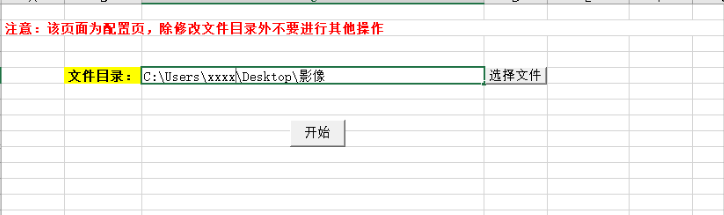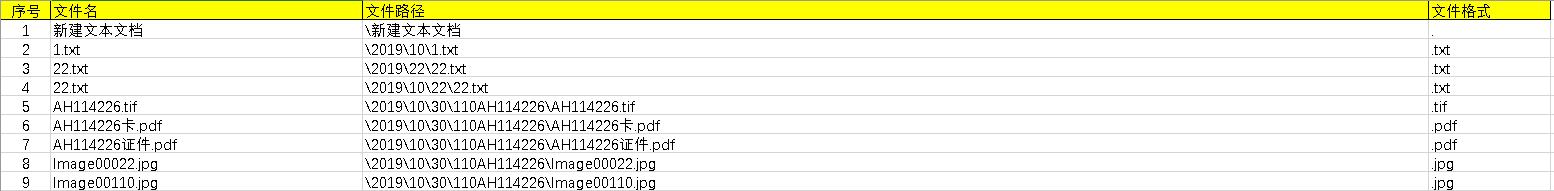• 通过设置文件设置读取指定路径下的文件，并指定读取内容示例，示例中详细注解可以参考。
• 将TXT文档的数据按照一定的格式写入Excel,并跟据日期或者特定条件可以查询数据，并自动绘制曲线图！不含任何空间，纯代代码，方便移植。
• ## VBA读写文本文件

万次阅读 2019-05-11 16:34:13
直接采用Open函数+Line input命令 Private Sub txt_read() Dim txt As String Open "D:\dzh.txt" For ... '对文件做任何 I/O 操作之前都必须先打开文件。Open 语句分配一个缓冲区供文件进行 I/O 之用， ...
直接采用Open函数+Line input命令
  Private Sub txt_read()
Dim txt As String
Open "D:\dzh.txt" For Input As #1 '
'对文件做任何 I/O 操作之前都必须先打开文件。Open 语句分配一个缓冲区供文件进行 I/O 之用，
'并决定缓冲区所使用的访问方式。
'打开文件作为数据输入用，文件号为#1
Do While Not EOF(1)
Line Input #1, txt  '从已打开的顺序文件中读出一行并将它分配给 String 变量
'Line Input # 语句一次只从文件中读出一个字符，直到遇到回车符 (Chr(13))
'或回车–换行符 (Chr(13) + Chr(10)) 为止。回车–换行符将被跳过，而不会被附加到字符串上
MsgBox txt
Loop
Close #1
End Sub
-----------------------------------------------------------------------------------------------
1、Open 语句
打开方式有
Input：以读取方式打开。
Output：以写入方式打开。
Append：以追加方式，即添加内容到文件末尾打开。
Binary：以二进制方式打开。
Random：以随机方式打开，如果未指定方式，则以 Random 方式打开文件。
Open pathname For mode As [#]filenumber
Open "c:\a.txt" For Output As #1
filenumber  是一个有效的文件号，范围在 1 到 511 之间。
如果 pathname 指定的文件不存在，那么，在用 Append、Binary、Output、或 Random 方式打开文件时，可以建立这一文件。
2、Close 语句
语法：Close [filenumberlist]
filenumberlist 参数为一个或多个文件号，若省略 filenumberlist，则将关闭 Open 语句打开的所有活动文件。
说明：打开文件后，必须在使用完后关闭文件。

3、EOF 函数
语法：EOF(filenumber)
功能：返回一个 Integer，它包含 Boolean 值 True，表明已经到达为 Random 或顺序 Input 打开的文件的结尾。
Open "c:\a.txt"  For Input As #1 ‘以读入方式打开c:\a.txt
Do While Not EOF(1)  '未到达结尾
Line Input #1, s1     '读入一行，放入s1变量中
Loop
Close #1

展开全文• vba完整的例子，有用户窗口操作，选择文件操作，读取文件，进行逻辑计算，获得结果，输出到excel表中。贪心算法，欧几里得定律。
• 除了使用专门的应用程序打开文件之外，你如果也想要创建VBA过程能够打开其它类型的文件并使用它们的内容的话，你就应该学习一些关于被称为低级别的文件I/O（input/output）。 接下来关于顺序，随机和二进制文件的...
我们知道使用VBA打开一个电子表格指令，例如指令： Application.Workbooks.Open Filename:= "C:\Excel\Report.xls"
打开位于文件夹C:\Excel里面的文件Report.xls。除了使用专门的应用程序打开文件之外，你如果也想要创建VBA过程能够打开其它类型的文件并使用它们的内容的话，你就应该学习一些关于被称为低级别的文件I/O（input/output）。
接下来关于顺序，随机和二进制文件的章节将会带你直接接触你的数据。
文件的访问类型
计算机使用的文件类型有三种：
①顺序访问文件是指按储存相同的顺序找回数据的文件。例如以CSV格式（逗号分割文本），TXT格式（以Tab键分割的文本）或者PRN格式（以空格分隔的文本）储存的文件。顺序文件访问经常用来写文本文件，例如错误日志，参数设定和报告。顺序文件有下列模式：Input,Output和Append。模式决定了文件打开后你如何使用它。
②随机访问文件是文本文件，它的数据以同等长度储存并在一个以逗号分割的区域了。随机访问文件只有一个模式——Random
③二进制访问文件是图形文件和其它非文本文件。二进制文件只能够在Binary模式下访问。
展开全文Excel
• 3.通过在工作表<式样.xlsx>中点击按钮来选择CSV文件读取其中的数据，并把相应的数据填入到当前工作表的指定位置。 **举例说明**： 如果选择读取的是110.csv文件，则需要将数据填入<式样.xlsx>中的B~F列中，...c++ c语言 perl 开发语言
• 打开一个Excel文件，选择菜单栏上的"Tools"选项->Macro->Visual Basic Editor，打开VBA编辑器。 在Modules文件夹下的csMain文件中，写下如下VB格式的代码：
 打开一个Excel文件，选择菜单栏上的"Tools"选项->Macro->Visual Basic Editor，打开VBA编辑器。
在Modules文件夹下的csMain文件中，写下如下VB格式的代码：

Public Sub Source做成()

'声明Excel相关
Dim xlApp As Excel.Application
Dim xlBook As Excel.Workbook

Set xlApp = New Excel.Application
'获取指定excel文件
Set xlBook = xlApp.Workbooks.Open("C:\test.xls")

Dim sheet As Excel.Worksheet
'获取指定sheet
Set sheet = xlBook.Worksheets(2)

Dim ss As String
'获取指定单元格的内容
ss = sheet.Cells(2, 2)

'内容显示
MsgBox (ss)

End Sub


展开全文excel
• 在EXCEL中使用VBA读取PDF文件的总页数，大大减少重复工作量。
• 开始参考了 VBA获取某文件夹下所有文件和子文件目录的文件中的代码，按照此方式获取的结果有问题。 问题1 无法获取目录名中包含“.”的子目录 '-- 获得所有子目录 Do Until i > k f = Dir(file(i), vbDirectory)...
公司运营部门需要把影像资料梳理一遍，文件目录特别多，文件量也大，大概40多个G。自己写了一个读取目录下所有子文件的脚本 开始参考了 VBA获取某文件夹下所有文件和子文件目录的文件中的代码，按照此方式获取的结果有问题。 问题1 无法获取目录名中包含“.”的子目录
'-- 获得所有子目录
Do Until i > k
f = Dir(file(i), vbDirectory)
Do Until f = ""
If InStr(f, ".") = 0 Then
k = k + 1
ReDim Preserve file(1 To k)
file(k) = file(i) & f & "\"
End If
f = Dir
Loop
i = i + 1
Loop

代码中使用InStr(f, “.”) = 0 判断，只要名字中包含"."就按照文件处理
问题2 无法获取扩展名为空的文件
'-- 获得所有子目录下的所有文件
For i = 1 To k
f = Dir(file(i) & "*.*")    '通配符*.*表示所有文件，*.xlsx Excel文件
Do Until f = ""
'Range("a" & x) = f
x = x + 1
f = Dir
Loop
Next

于是，自己实现了一个支持文件夹名称带“.”或文件名不带扩展名的。 实现过程 新建一个文件，在sheet1中增加两个按钮，一个用来选取文件夹，一个用来执行查询
选择文件脚本
Option Explicit
Sub 打开文件夹()

With Application.FileDialog(msoFileDialogFolderPicker)
If .Show = -1 Then
Worksheets("Sheet1").Range("C5").Value = .SelectedItems(1)
End If
End With

End Sub


执行脚本

Sub 按钮1_Click()

On Error Resume Next

Dim folderObj As Object
Dim currFolder
Dim fdCnt As Integer

Dim sDir As String

Dim dirExist, f As String
Dim file(), subFolder(), allfd() As String
Dim fileNum, k, x, idx, i, j, listNum
Dim threeDir As String

fileNum = 1
x = 1
k = 1
j = 0
i = 1

sDir = Worksheets("Sheet1").Range("C5").Value

'=== 0.清除数据=============================================
Sheet2.UsedRange.Clear

Worksheets("Sheet2").Range("A1").Value = "序号"
Worksheets("Sheet2").Range("C1").Value = "文件名"
Worksheets("Sheet2").Range("D1").Value = "文件路径"
Worksheets("Sheet2").Range("E1").Value = "文件格式"
Worksheets("Sheet2").Range("E1").Interior.Color = RGB(255, 255, 0)
Worksheets("Sheet2").Range("A1").Interior.Color = RGB(255, 255, 0)
Worksheets("Sheet2").Range("C1").Interior.Color = RGB(255, 255, 0)
Worksheets("Sheet2").Range("D1").Interior.Color = RGB(255, 255, 0)
Worksheets("Sheet2").Range("E1").Borders.LineStyle = xlContinuous
Worksheets("Sheet2").Range("A1").Borders.LineStyle = xlContinuous
Worksheets("Sheet2").Range("C1").Borders.LineStyle = xlContinuous
Worksheets("Sheet2").Range("D1").Borders.LineStyle = xlContinuous

'=== 1.判断选择的文件夹是否有效===============================

dirExist = dir(sDir, vbDirectory)
If dirExist = "" Then
MsgBox ("选择的文件夹无效")
Exit Sub
End If

'=== 2.获取所有子目录======================================

ReDim subFolder(1 To i)

subFolder(1) = sDir & "\"
f = dir(subFolder(1), vbDirectory)
Do Until f = ""
If f <> "." And f <> ".." Then
If (GetAttr(subFolder(1) & f) And vbDirectory) = 16 Then
'Worksheets("Sheet3").Range("A" & k).Value = subFolder(1) & f & "\"
k = k + 1
ReDim Preserve subFolder(1 To k)
subFolder(k) = subFolder(1) & f & "\"
End If
End If
f = dir
Loop
i = i + 1

Dim tmp As Integer
tmp = 0

For Each fd In subFolder
tmp = tmp + 1
ReDim Preserve allfd(1 To tmp)
i = 1
k = 1
Erase file
ReDim file(1 To i)
file(i) = fd
allfd(tmp) = fd
Worksheets("Sheet3").Range("B" & tmp).Value = allfd(tmp)

If subFolder(1) = file(i) Then
f = dir
i = i + 1
Else

Do Until i > k
f = dir(file(i), vbDirectory)
Do Until f = ""
If f <> "." And f <> ".." Then
If (GetAttr(file(i) & f) And vbDirectory) = 16 Then
k = k + 1
ReDim Preserve file(1 To k)
file(k) = file(i) & f & "\"
tmp = tmp + 1
ReDim Preserve allfd(1 To tmp)
allfd(tmp) = file(i) & f & "\"
' Worksheets("Sheet3").Range("B" & tmp).Value = allfd(tmp)
End If
End If
f = dir
Loop
i = i + 1
Loop
End If
Next

'=== 3.获取所有子目录下的文件======================================
'
Dim threeStr As String

x = 2
idx = 1
For i = 1 To tmp

f = dir(allfd(i) & "*.*")
Do Until f = ""
Worksheets("Sheet2").Range("A" & x).Value = idx
Worksheets("Sheet2").Range("C" & x).Value = f
Worksheets("Sheet2").Range("D" & x).Value = Replace(allfd(i), sDir, "") & f
'Worksheets("Sheet2").Range("E" & x).Value = getFileType(f)

'Worksheets("Sheet2").Range("B" & x).NumberFormatLocal = "@"
'Worksheets("Sheet2").Range("B" & x).Value = getToubaodanHao(sDir, allfd(i))

f = dir
x = x + 1
idx = idx + 1
Loop

Next

End Sub


最终效果：参考
W3CSchool VBA教程VBA获取某文件夹下所有文件和子文件目录的文件VBA 快速入门
展开全文• 文本文件可以有不同编码格式（ANSI，UTF8，UTF16 等等），文本编辑器可以自动识别相应的编码格式，例如下面两个文件内容完全相同，但是文件格式却不同。 Sub ReadUTFTxt() Dim objStream, strData Set objStream ...UTF ADODB 字符集 ANSI
• 上一篇文章我们提到：更好的处理方式就是读取将每一行，按照空格再分为不同的单元格 代码实现如下： Sub bb() Dim a, b, k%, i%, j%, q% Open "a.txt" For Input As #1 a = Split(StrConv(InputB(LOF(1), 1), vb...StrConv
• 1、直接采用Open函数+Line input命令 Private Sub txt_read() Dim txt As String ... '对文件做任何 I/O 操作之前都必须先打开文件。Open 语句分配一个缓冲区供文件进行 I/O 之用， '并决定缓冲区所使用windows
• Shell "RUNDLL32.EXE URL.DLL,FileProtocolHandler " & myfile, vbMaximizedFocus ' myfile是PDF文件完整路径
• For Output As #2 k = 0 Do While Not EOF(1) Line Input #1, txtLine '读取一行的数据，一直读到文件尾 k = k + 1 If k = 1 Then For I = 0 To UBound(Split(txtLine, ",")) Str1 = Split(txtLine, ",")(I) If Str1...
• VBA使用FileSystemObject将读取或写入文本文件
• 案列：现需要按条件汇总一个文件夹下的多个Excel文件中的某列数据到汇总表格中，文件夹中的所有Excel文件都是基于一个模板，只是数据不同。 所有的Excel文件结构： 库存组织:XXX 货主类型:XXX 货主:...
• VBA 读取/写入文本文件  2011-06-28 10:53:58| 分类： Excel自动化 | 标签：读入 文本  |举报 |字号 订阅 1、Open 语句  打开方式有  Input：以读取方式打开。 Output：以写入方式...
• 项目背景近期接到一个工作任务，在运行设备中，会定期生成数据记录文件（txt 格式），我需要将这个文件中的 1000 个数据导入到 Excel 中，通过公式计算，得到需要的另外两列数据，将这两列数据作为数据源...
• '返回对应路径下第一个符合*.csv的文件名称 If wechatFile = "" Then MsgBox "请将微信商户号下载的订单文件放至目录" & currentFileDirectory End If Do While wechatFile <> "" '当返回的...
• VBA按行读取TXT文本文件 文件格式 窗口上添加一个文本控件 TextBox1 属性设置 MultiLine ：True ScrollBars ：3 - fmScrollBarsBoth 代码如下： Private Sub CommandButton1_Click() Dim strTxt() As ...
• '打开文件对话框返回的文件名，是一个全路径文件名，其值也可能是False，因此类型为Variant Dim i As Integer File = Application.GetOpenFilename("Excel 文件,*.xls;*.xlsx;*.xlsm") '调用Windows打开文件...excel
• ﻿﻿XML的简单使其易于在任何应用程序中读写数据，这使XML很快成为数据交换的唯一公共语言，虽然不同的应用软件支持其它的数据交换格式，但不久之后他们都...下面我们来学习一下用VBA如何读取XML文件和输出XML文件1....XML
• ## Excel-VBA文件操作

千次阅读 2018-10-05 02:16:29
• 通过Java读取指定行列的数据，请用eclipse平台打开。实现思想详见我的博客。
• Sub OptionalFeatureLicense() Dim Fs As Object, Ft As Object, S As String Set Fs = CreateObject("Scripting.FileSystemObject") Set d = CreateObject("scripting.dictionary") filePath = Th...
• VBA生成或读取指定字符集的文本文件VBA做工具的过程中，遇见这样一个问题。使用FSO方式或者直接OPEN文件方式，生成的文本文件采用的字符集为当前操作系统默认字符集，不能选择字符集类型。这样的文件作为应用...ADO
• Open 文件名 for 打开方式 as 文件编号 打开方式:Input ：只能...读取txt文件内容方法input：从文件读取指定数量的字符。Input #：把数据读出放在变量里，变量用逗号分隔Line Input #：取出完整的一行 向文件中写...
• 如果你需要检查某文件是否能够存在某磁盘上，那么你应该按照下述方式使用FileLen函数： FileLen(文件路径名) FileLen函数一字节方式返回文件的大小。如果该文件已打开，那么VB将返回该文件最后一个保存时的大小。 ...excel
• iamlaosong文 文件的日期时间分创建时间和修改时间，文件一旦创建，创建...1、取文件的修改时间VBA有专门的函数FileDateTime，如下面的语句： PicName = ThisWorkbook.Path &amp; "\YiCode.bmp" ......